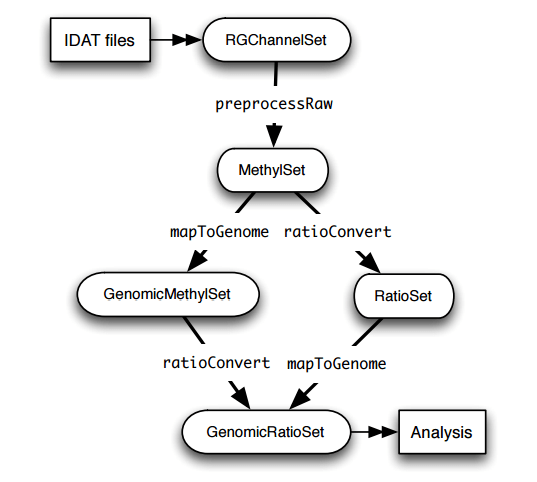## 甲基化芯片分析-minfi包

`ChAMP`包的读入数据也是利用`minfi`包的方法，所以两者在这个点上是一致的。`minfi包`的分析流程结构如下（取自minfi文档）：`IDAT files``GenomicRatioSet`这个可用于下游分析（差异分析）的对象，主要经过`RGChannelSet`(probe level)，`MethylSet`(CpG locus level)，`GenomicMethylSet`(mapped to genome) or `RatioSet`(Beta and/or M (logratio of Beta))这些步骤

1. 读入IDAT文件，也想`ChAMP`包一样准备好一个sample_sheet.csv的文件，放在R的当前目录下（该目录下只能有其一个csv文件）

``````library(minfi)
RGset <- read.metharray.exp(targets = targets)
pd <- pData(RGset)
annotation(RGset)    #查看所会用到的注释文件有哪些
``````

如果没有`sample_sheet.csv`文件也是可以读入IDAT数据的，只是会没有一些样品信息

2. 对读入后的原始数据进行预处理，bioconductor的minfi处理甲基化数据这篇文章大概列了几种预处理的函数，这里就用Illumina的标准处理（类似于 performed by Genome Studio）

``````pRGset <- preprocessIllumina(RGset)
mRGset <- mapToGenome(pRGset)
``````

除了用这个外，还可以自己定义过滤标准，类似于`ChAMP`包封装的那些标准，自己用R代码来过滤，比如https://github.com/wkl1990/illumina-450K-analysis/blob/master/R/450K_pipeline.R，这样好处是直观并且了解了过滤过程

还有一些标准化函数，如：`preprocessSWAN``preprocessQuantile`以及`preprocessFunnorm`，（看着眼熟，原来都整合在`ChAMP`包里了）

3. 接着转化为`GenomicRatioSet`对象

``````dat <- ratioConvert(mRGset,type="Illumina")
``````
4. 最后设置个分组矩阵，然后就是差异甲基化位点分析，比如用下`limma`

``````library(limma)
bate <- getBeta(dat)
design <-model.matrix(~pd\$Sample_Group)
fit <- lmFit(bate, design)
fit2 <- eBayes(fit, trend=TRUE)
output <- topTable(fit2, coef=2,n=Inf)
``````

当然minfi中差异分析则不是用limma的，而是`dmpFinder`函数，下面是文档的例子

`````` dmp <- dmpFinder(mset, pheno=pd\$Sample_Group, type="categorical")
``````
5. 如果是Differential Methylation Regions分析，则使用`bumphunter`函数

``````library(doParallel)
detectCores()
registerDoParallel(cores = 6)
design <-model.matrix(~pd\$Sample_Group)
res <- bumphunter(dat, design, cutoff=0.1)
``````